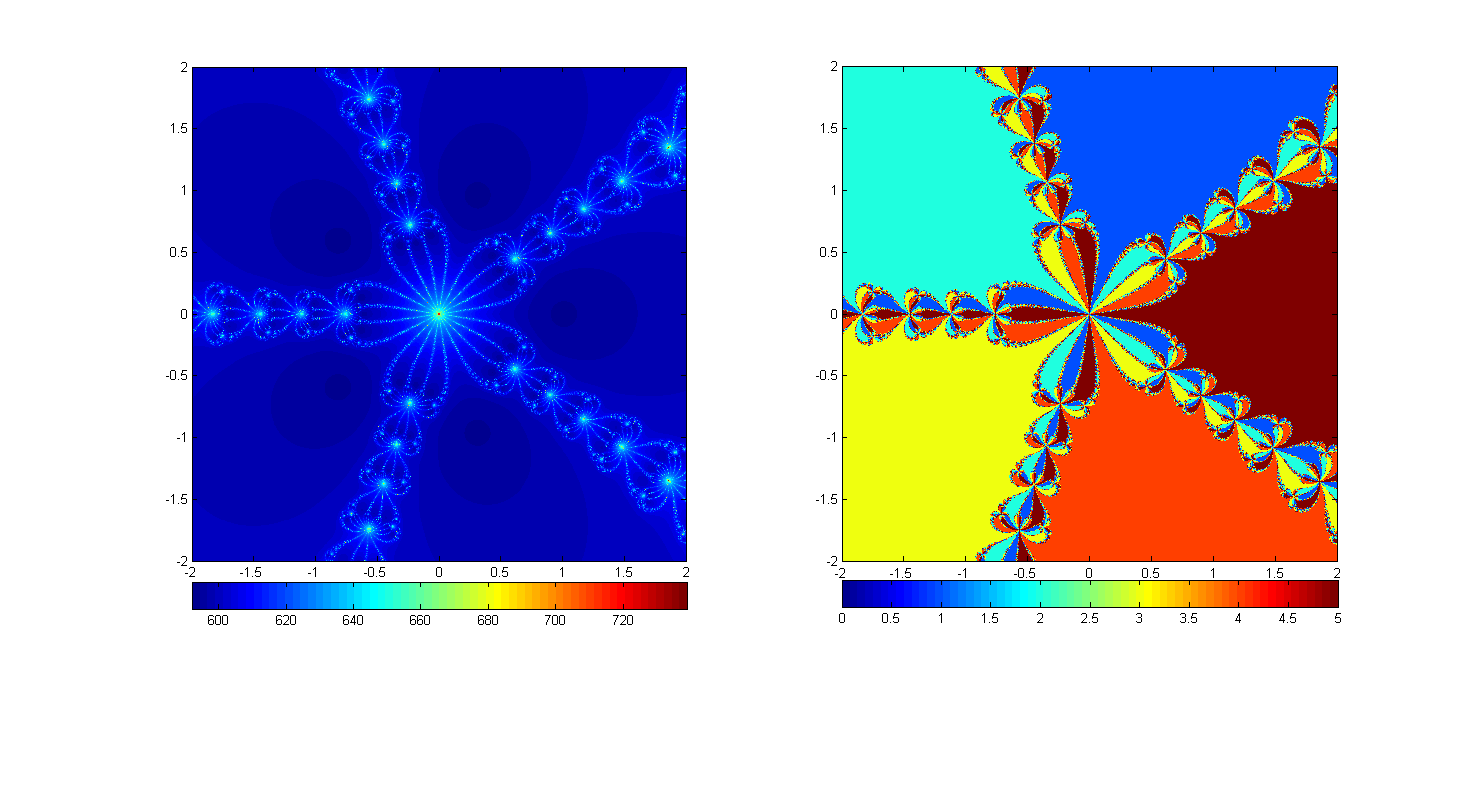## General info

• Syllabus
• Lectures: Tu-Th 11:40-1:15pm, McHenry 1270.
• Office hours: by appointment.
• Main reference: "Complex Analysis" by Stein and Shakarchi
• Other good references:
• Lecture Notes: I will type lecture notes when the material differs substantially from the main reference. You may also find Alvin Jin's live-tex'd lecture notes here (thanks Alvin !). If all else fails and you missed a class and would like to catch up, email me and I will send you scanned lecture notes.

## Lectures

• 4/4 - Lecture 1: Preliminaries (Ch1, Sec. 1, 2.1, 2.2). Field properties, conjugation, norm. Convergence, completeness. The exponential function, the law of exponents and its consequences. Polar forms, nth roots. Set topology on the complex plane. Continuous functions, holomorphic functions, examples, Cauchy-Riemann equations.
• 4/6 - Lecture 2: Preliminaries (Ch1, Sec. 2.3, 3). Power series, Hadamard's formula and the disc of convergence. Smoothness inside the disc of convergence. Analyticity. Curves and their parameterization/orientation, integration along curves, length, ML estimates, primitives, complex FTC, holomorphic functions with zero derivative are constant.
• 4/11 - Lecture 3: Cauchy integral theorem/formula (Ch2 up to thm 4.1). Goursat's theorem on triangles, squares. Construction of primitives and Cauchy's theorem on toy contours. Applications to computation of integrals (fourier transform of a gaussian, oscillatory integral with a pole). Cauchy integral formula.
• 4/13 - Lecture 4: Cauchy integral theorem/formula: consequences. Existence of higher-order derivative, local power series expansions, Cauchy estimates. Liouville, fund. thm. of algebra. Identity theorem and analytic extension. Morera and consequences of normal convergence. Schwartz reflection principle. (Skipped: 5.3, 5.5)
• 4/18 - Lecture 5: Isolated Singularities. Residue formula and examples. (Ch3, Sec. 1-2) The three types of isolated singularities. Residue formula. Computation of integrals: real, rational functions with non-real poles; integrals with periodic pole structure; trigonometric integrals (e.g., Gamelin, Ch. VII.3).
• 4/20 - Lecture 6: Trichotomy of isolated singularities. Argument principle, Rouché's and Hurwitz' theorems. Riemann's theorem on removable singularities, Casorati-Weiestrass (see Ch3, Sec 3). Here are notes for the remaining theorems.
• 4/25 - Lecture 7: Open mapping an inverse functions. Local existence of analytic logs and roots. Critical points and local behavior near them. Some lecture notes are here.
• 4/27 - Lecture 8: The extended complex plane, rational functions and Möbius transformations.
• 5/2 - Lecture 9: Möbius transformations: Zoology of the conformal automorphisms of the extended complex plane and the unit disc.
• 5/4 - Lecture 10: Simple connectedness and consequences. Conformal equivalences.
• 5/9 - Lecture 11: Montel's theorem and the Riemann Mapping Theorem
• 5/11 - Lecture 12: Schwarz-Christoffel: mapping onto polygonal domains.
• 5/16 - Lecture 13: Examples of mapping onto polygons. Entire functions, density of zeros and infinite products (preliminaries). (Stein, Ch5 up to section 3.1 included)
• 5/18 - Lecture 14: Weierstrass products, Hadamard's factorization theorem.
• 5/23 - Lecture 15: The Gamma function. Functional equation for the theta function (prep. for Zeta). (Ch6, sec. 1)
• 5/25 - Lecture 16: The Zeta function. Functional relations, meromorphic continuation, Euler's identity. Study of the zeros, trivial zeros, absence of zeros on the set {x=1}.
• 5/30 - Lecture 17: The prime number theorem. (Stein, Ch7, sec. 1.1 onwards)
• 6/5 - Lecture 18: Introduction to complex dynamics - 1/3.
• 6/6 - Lecture 19: Introduction to complex dynamics - 2/3 - Julia and Fatou.
• 6/8 - Lecture 20: Introduction to complex dynamics - 3/3 - Connectedness of Julia sets and the Mandelbrot set.

## Problem sets

• 4/6 - HW1 - due 4/13.
• 4/13 - HW2 - due 4/20.
• 4/20 - HW3 - due 4/27.
• 5/1 - HW4 - due 5/9.
• 5/9 - HW5 - due 5/16.
• 5/19 - HW6 - due 5/26.